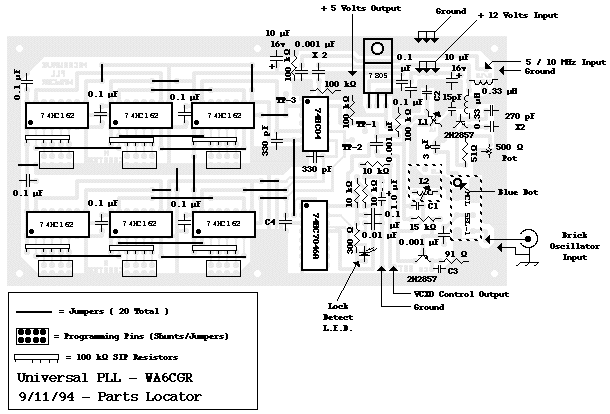Dave Glawson - WA6CGR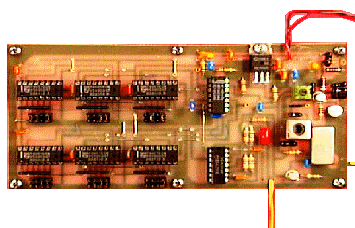Background

One of the more critical factors involved in microwave SSB communications is knowing where your frequency is while operating in the field. The uncertainty of frequency only adds to the frustration one experiences. This, while attempting to communicate with other stations who are also concerned with antenna direction, band conditions, weather, equipment performance, power supplies and other factors that make life so enjoyable during microwave contests.

The system described in this article is an upgrade to a previous project that I produced to stabalize microwave "brick" oscillators. This Phase Locked Loop (PLL) system and the accompanying Voltage Controlled Crystal Oscillator (VCXO) circuit are suitable for use with any microwave system.

Microwave "Brick" Oscillators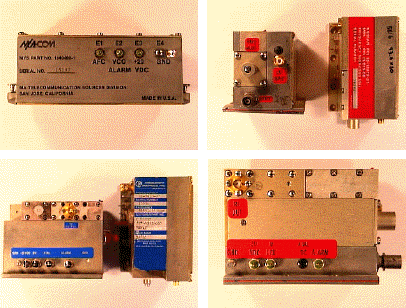The basic system consists of a 5 or 10 MHz Oven Controlled Crystal Oscillator (OCXO) as the frequency reference, two programmable BCD dividers, a mixer, a frequency multiplier, and a phase detector with lock detect.

The system is "Universal" in that it is designed to be used with 5th or 7th overtone crystal oscillators in the range of 50 to 120 MHz which is in the range of most microwave Local Oscillator (LO) circuits before multiplying.

As most microwave transverter systems use 28 or 144 MHz transceivers as the Intermediate Frequency (IF) radio, this system is also well suited to phase lock the 116 MHz oscillator used in the 2 meter to 10 meter converter.

The circuit is based on a hi-speed CMOS 74HC7046AN low power phase lock loop chip used as the phase comparator and lock detect circuit. The 74HC7046AN contains both type 1 and type 2 phase detectors, and a VCO circuit that is not used. The type 1 detector demands that the two input signals have a 50% duty cycle and the type 2 detector uses the leading edge of each of the squared input signals and the duty cycle is irrelevant. This system uses the type 2 phase detector.

The 74HC7046AN produces a linear voltage output of 0-5 volts depending on the phase difference of the input signals. This analog signal is then fed through a low pass filter to set the loop response time as well as filter out the reference divider frequency. The latter function is not as critical as in most PLL systems that are based on locking a Voltage Controlled Oscillator (VCO) due to the limited frequency range that a crystal oscillator can be pulled.

Loop Response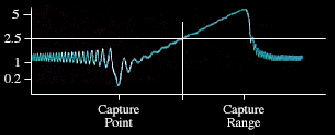The 74HC7046AN is useful with up to 24 MHz input signals.

Circuit Description

The stability of the system is dependant upon the stability of the reference oscillator. The better your reference oscillator is, the more stable your final microwave signal will be. The 5 MHz OCXO's that I have been using, have a typical stability of 0.01 ppm (parts-per-million) or 100 cycles at 10 GHz.

Either a 5 or 10 MHz reference oscillator can be used with this circuit with no change in components. The frequency you use will be entered into the computer program to determine the divider programming. Reference oscillators suitable for this system can usually be found at Swap Meets or they can be purchased from various manufacturers.

The cost from the manufacturer is quite high in amateur terms (in the range of \$150-\$250) so it pays to spend some time searching for one. This system will use reference oscillators with either a sine or square wave output. It is possible to use a high quality Temperature Compensated Crystal Oscillator (TCXO), but the stability of commercially available units are usually less than desireable (1 ppm or worse, 1 ppm = 10 kHz @ 10 GHz).

Reference Oscillators - OCXO's and TCXO'sThe reference oscillator of 5 or 10 MHz is changed to an HCMOS compatible squarewave through two 74HC04 HCMOS gates. The first gate is biased linear and provides enough gain to saturate the next gate. This HCMOS signal is than fed directly to the reference frequency divider (the 'M' divider) and then it is buffered and connected to the input of a 2N2857 multiplier. This multiplier can be tuned from 50 to 120 MHz by selecting the capacitor across the collector coil. Use a 20 pF capacitor for 50-70 MHz, an 18 pF capacitor for 70-90 Mhz, a 15 pF capacitor for 90-110 MHz and a 12 pF capacitor for 110-120 MHz.

The multiplier output is coupled to a Mini-Circuits SBL-1 double balanced mixer. The input of the mixer comes from the external overtone oscillator. The one I have included with this article has an output amplified by a Mini-Circuits MAR-8 MMIC to provide an adequate signal from the sampled output of the overtone oscillator circuit and to provide a sgnificant amount of reverse isolation from the overtone oscillator to prevent the difference frequency (overtone oscillator minus the multiplier frequency) from modulating the overtone oscillator.

As an example, in my latest X-Band radio, I use an overtone oscillator frequency of 100.2353 MHz (when multiplied by 102 becomes 10.224 GHz. This plus 144 MHz = 10.368 GHz).

Therefore, I feed the mixer with a signal from the overtone oscillator of 100.2353 MHz and I use a 15 pF capacitor accross the multiplier coil for 100 MHz and I get a difference frequency of 235.3 kHz.

The output of the mixer is the difference frequency (overtone oscillator minus the multiplier frequency) which is amplified by another 2N2857 whose collector is tuned to the difference frequency (235.3 kHz) or 0.047 �F accross the 10�H varible inductor (see schematic), and is squared in the same fashion as the OCXO signal, with two 74HC04 gates. The output of this amplifier supplies the signal for the difference or 'N' divider.

The system can be easily adjusted by connecting an oscilloscope to the collector of the difference amplifier (TP-1) and tune L1, L2, and R1 for maximum sine wave amplitude at the difference frequency.

Programming of the presettable BCD synchronous counters is accomplished by running the computer program listed at the end of this article. The program is written for either 5 or 10 MHz reference oscillators. After running the program, enter your brick oscillator frequency and your reference frequency. The program will calculate the division ratios for the "M" and "N" counters. "M" is the number by which the reference frequency is divided and "N" is the number by which the difference frequency is divided.

Each counter chain consists of three 74HC162 BCD presettable synchronous counter chips, each with the 4 BCD programming lines. Each programming line is automatically pulled high (logic "1") by a 100 k resistor and is made low (logic "0") by placing a jumper to ground.

The computer program provides the programming information to preset the counters. In the example program run, I chose a brick frequency of 100.2353 MHz and a Reference oscillator frequency of 5 MHz. The program provided the following information:

Example 1

-----------------------------------------------------------
Run
Enter Brick Frequency (Return for 103.0794) 100.2353
Enter Reference Oscillator Frequency (Return for 5) 5
Brick Oscillator Frequency= 100.2353 MHz
Reference Oscillator Frequency= 5 MHz
Difference Frequency= .2352981567382812 MHz
These numbers represent the actual division ratios.
'M' 'N' Calculated Difference Frequency in MHz
85 4 .2352941176470588
These numbers represent the counter preset numbers.
'M' 'N'
915 996
Ok
-----------------------------------------------------------

The reference frequency of 5 MHz will be divided by 85 and the difference frequency of 235.29411 kHz will be divided by 4. 5 MHz / 85= .05882352941 MHz or 58.82352941 kHz. .23529411 / 4= .05882352941 MHz or 58.82352941 kHz.

The counters are then programmed as follows:
The"M" counter division ratio is "85". Subtract this number from 1000 and the resultant number is the BCD counter preset number "915".

The Most Significant Digit (M.S.D. or 100's Digit), U6, is then programmed for the BCD number "9" so all that is necessary is to ground pins 4 and 5 leaving pins 3 and 6 high (open or logic "1"). U5 (the 10's Digit) is programmed with a BCD "1" so ground pins 4, 5 and 6 leaving pin 3 high. And finally, U4 the Least Significant Digit (L.S.D. or 1's Digit) is programmed with a BCD "5" so ground pins 3 and 5 leaving pins 4 and 6 high. The resultant BCD codes for U4, U5 and U6 are "1010", "1000", and "1001" respectively.

The "N" counter is programmed in the same way with the resultant BCD codes for U1, U2, and U3 being "0110", "1001", and "1001" respectively representing BCD "996".

The computer output using the same brick oscillator frequency but a reference frequency of 10 MHz would be:

Example 2

-----------------------------------------------------------
Run
Enter Brick Frequency (Return for 103.0794) 100.2353 Enter Reference Oscillator
Frequency (Return for 10) 10 Brick Oscillator Frequency= 100.2353 MHz
Reference Oscillator Frequency= 10 MHz
Difference Frequency= .2352981567382812 MHz
These numbers represent the actual division ratios.
'M' 'N' Calculated Difference Frequency in MHz
85 2 .2352941176470588
These numbers represent the counter preset numbers.
'M' 'N'
915 998
Ok
-----------------------------------------------------------

So the "M"counter (U4, U5, and U6) would be programmed "1010", "1000", and "1001" respectively representing a BCD "915" and the "N" counter (U1, U2, and U3) would be programmed the same as before with "0001", "1001", and "1001" or BCD "998".

The only modification to your existing microwave brick oscillator assembly, is to solder one end of a 3 pF capacitor to the crystal trimmer inside the brick. Attach an MV-2111 (or similar) varicap from the other end of the 3 pF capacitor to ground. At the junction of the varicap and the 3 pF capacitor attach a 10k� resitor from this point to a feed-thru capacitor usually supplied with the brick for "AFC" purposes. This modification is shown on the schematic in the area marked "Inside Brick".

Set-up is simple. Install the 3 pF capacitor, the varicap, and the 10k� resistor as discussed. Warm up the oscillator assembly with +1.5 volts connected to the 10k� resistor. Tune the crystal trimmer in the brick to center frequency. Then after aligning the P.L.L. circuit as discussed, attach the crystal monitor output of your brick oscillator or VCXO to the R.F. port of the SBL-1 mixer of the P.L.L. circuit and the frequency should lock immediately.

The total current drawn from the P.L.L. circuit excluding the 5 MHz reference oscillator I used, was 150mA at 12 volts.

If you have operated microwave SSB systems in the past and have been victim of drifting oscillators or don't often have access to sophisticated test equipment moments before going to the hilltop for a contest, then the performance of this system will be a welcome relief. I have included an oscillator circuit compatible with this PLL system for use with 5th or 7th overtone crystals in the 50 - 120 MHz range. The component values are listed on the schematic diagram of the oscillator. I have also included a suggested layout for the oscillator.

The thought of being within 100 Hz at 10.368 GHz within a few minutes of applying power to the radio should generate enough enthusiasm to persue this project. See below for board and kit availability.

Here is a sample of actual audio from Jack - N6XQ at 504 miles (811 km) away (DL27QP) talking to
Dave - WA6CGR (DM04RD): N6XQ.au (231 K) AU format audio file.

Use NAPlayer for Windows or Sound Machine on the Macintosh.
Netscape 3.0 for both environments will play the sound without helper applications.

I would like to thank Chip Angle - N6CA, Terry Flach - WU6N and Bart Rowlett - WB6HQK for their technical assistance and insight on this project. I would also like to thank C.L. Houghton - WB6IGP and Kerry Banke - N6IZW for their original article prompting me to do this.

Program Listing

This program is written in Microsoft BASIC which runs on the Macintosh or IBM compatible.

5 CLS 10 DEFDBL A-Z 12 BF=0:RF=0:RO=0 20 DEFINT I 30 DIM P(13) 40 REM THIS PROGRAM CALCULATES THE DIVIDE BY "M" AND "N" COUNTER 50 INPUT "Enter Brick Frequency (Return for 103.0794) ",BF 52 IF BF=0 THEN BF=103.0794 55 PRINT 60 INPUT "Enter Reference Oscillator Frequency (Return for 10) ",RF 61 IF RF=0 THEN RF=10 62 RO=RF 65 PRINT 70 REM RH IS HARMONIC NUMBER BEING COMPARED 80 REM HD IS DIFFERENCE BETWEEN RH AND BF 90 REM M IS THE NUMBER BY WHICH THE REFERENCE IS DIVIDED 100 REM N IS THE NUMBER BY WHICH THE DIFFERENCE IS DIVIDED 110 REM S & U SET PRECISION OF DIVISION MATCH 112 IF RF=5 THEN RF=10 120 RH=CINT(BF/RF) 130 PRINT "Brick Oscillator Frequency= ";BF;"MHz" 135 PRINT 140 PRINT "Reference Oscillator Frequency= ";RO;"MHz" 145 PRINT 150 HD=ABS(BF-RF*RH) 160 PRINT "Difference Frequency= ";HD;"MHz" 162 IF RO=5 THEN RF=5 165 PRINT 170 IF RF*RH>BF THEN PRINT "Phase Sense Reverse" 175 PRINT "These numbers represent the actual division ratios.":PRINT 180 PRINT " 'M' ";" 'N'"; " Calculated Difference Frequency in MHz" 190 M=1 200 N=M*ABS(HD)/RF 210 S=ABS(RF*INT(N)/M-HD) 220 IF S<.00001 THEN PRINT M;INT(N);RF*INT(N)/M 225 NC=INT(N) 230 IF S<.00001 THEN 300 240 U=ABS(RF*INT(N+1)/M-HD) 250 IF U<.00001 THEN PRINT M;INT(N+1);RF*INT(N+1)/M 255 NC=INT(N+1) 260 IF U<.00001 THEN GOTO 295 270 M=M+1 280 GOTO 200 290 REM THIS CALC M CNTR PRESET FOR M=1 295 N = INT(N) +1 300 REM This is a modified version of the Brick oscillator program 310 REM written by C.L. Houghton - WB6IGP and Kerry Banke - N6IZW 320 REM 330 REM Modifications were written by Dave Glawson - WA6CGR 4/11/93 335 PRINT:PRINT "These numbers represent the M and N counter preset numbers":PRINT 340 PRINT " 'M' ";" 'N' " 350 PRINT (1000-M);(1000-NC) 360 END

Schematic Diagram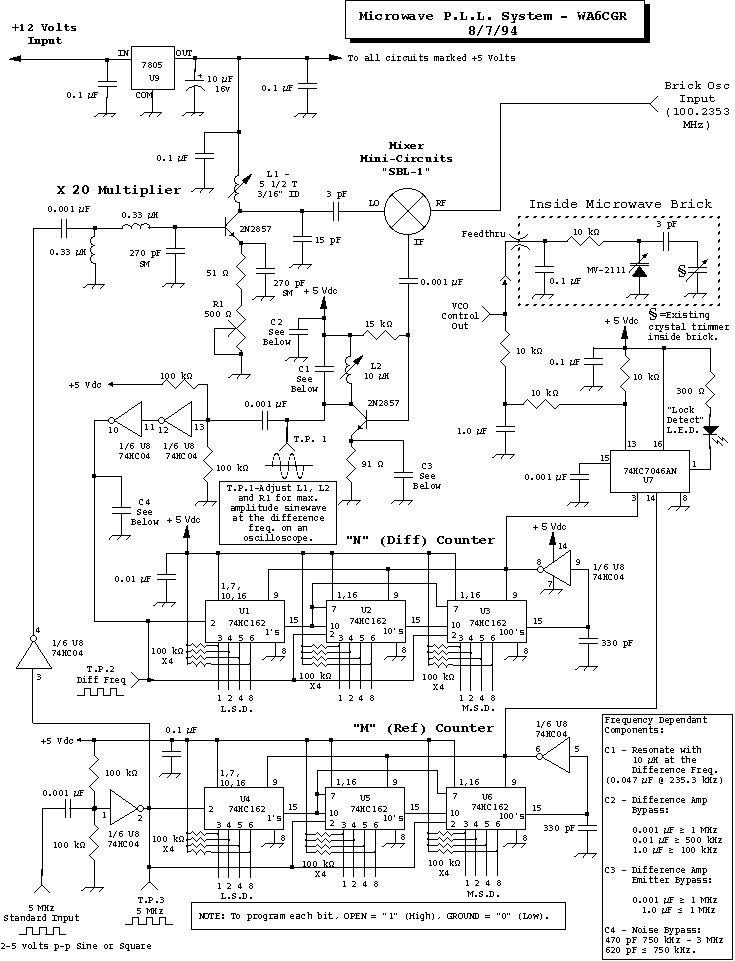Printed Circuit Board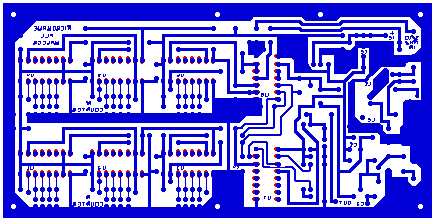Parts Locator